# Interpreting Graphs## Overview

Expect to see a number of questions on the SAT that deal with interpreting information presented graphically. In this section, we will review the following aspects of this topic:

• Drawing Conclusions from Graphs: Categorical Data
• Drawing Conclusions from Graphs: Continuous Data

Let’s look at each of these in more detail:

### Drawing Conclusions from Graphs: Categorical Data

Different data types result in different types of graphs. Categorical data includes non-numerical data. For example, here is a data table that shows fruit preferences for students in a class. Column 1 of the data set consists of the categories (the fruit). Column 2 of the data set is numerical. This is a categorical data set.

 Fruit Preferences Apples 12 Bananas 11 Oranges 8 Pineapples 5 Kiwis 3

Here is the bar graph of that data: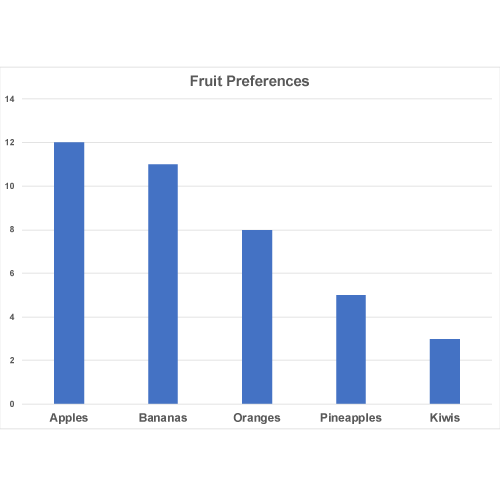One type of graph that you are likely to see is a line graph. What is a line graph? It usually starts with a data table. This table tracks the average number of cars sold each day of the week at a car dealership. Note that column 1 is categorical, the days of the week, while column 2 is numerical.

 Day Number of Cars Sold Monday 19 Tuesday 21 Wednesday 25 Thursday 24 Friday 33 Saturday 75 Sunday 80

Line graphs are useful for showing a trend over time. Here is a graph of the data.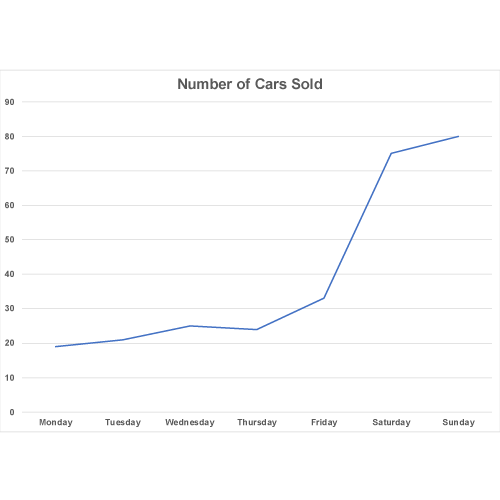The horizontal axis includes the days of the week, which corresponds to column 1 of the data table. The vertical axis is the number of cars sold, which corresponds to the other data column.

From this graph you can conclude the following:

• More cars are sold on Saturday and Sunday.
• The least number of cars are sold on Monday and Tuesday.

Expect to see a line graph with one or more questions that involve analyzing the graph.

 SAT Skill: Analyzing Graphs Example 1 A group of scientists is tracking the movements of a bear. The vertical axis tracks how far the bear is from the scientists’ camp. The horizontal axis tracks the time of day.Questions you might be asked about this graph: During what periods was the bear closest to the camp? What might be the reason that the bear came closest to the camp when it did? During what period of time was the bear farthest from the camp? What might account for this? These are the three places on the graph where the bear is closest to the camp (around 20 feet). Note the times of day. What do you notice? The bear comes closest to the camp around meal times for the campers.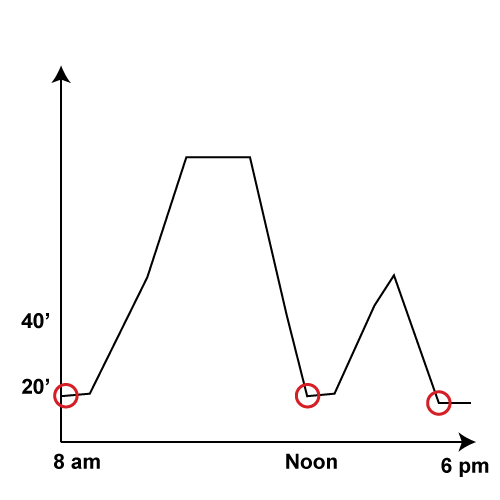This is the period when the bear is farthest away from the camp. The flat line indicates that the bear isn’t moving away from the camp and could indicate that the bear is resting or napping.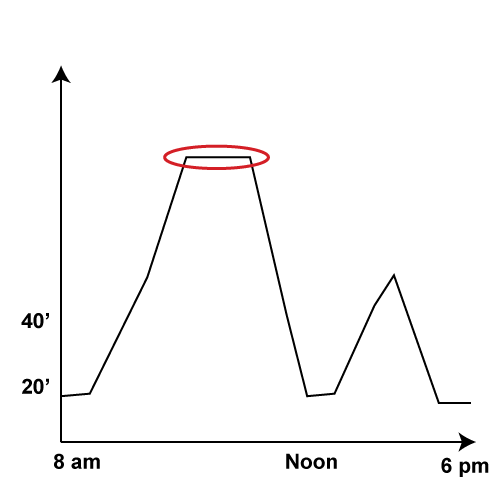Example 2 This graph shows the data for a three-hour round trip car ride . The vertical axis tracks the distance from home and the horizontal axis track the three hours. Which segment of the trip fits this description: “Having reached the destination, they took a half-hour break.”A “break” means that the car is no longer moving away from its starting point. That happens in the segment EF. And this section also accounts for roughly a half hour of elapsed time.

### Drawing Conclusions from Graphs: Continuous Data

Another data type you should be familiar with is continuous data. You’ll often see bivariate data (two-variable data) that consists of a set of measurements. Here is an example.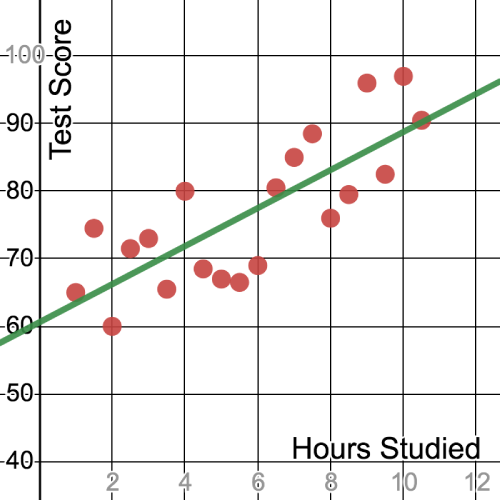This scatterplot shows the relationship between the number of hours studied and the results on a test for a number of students. What can you conclude from this graph?

The horizontal axis tracks the number of hours studied. The vertical axis represents the test score. A scatterplot like this shows a functional relationship between the two variables. This scatterplot shows a linear relationship and the green line is known as the line of best fit.

From this graph we can conclude that as the number of hours increased, so did the test score.

 SAT Skill: Analyzing Graphs Example 1 This scatterplot shows the correlation between the prices of two different stocks. Describe the relationship.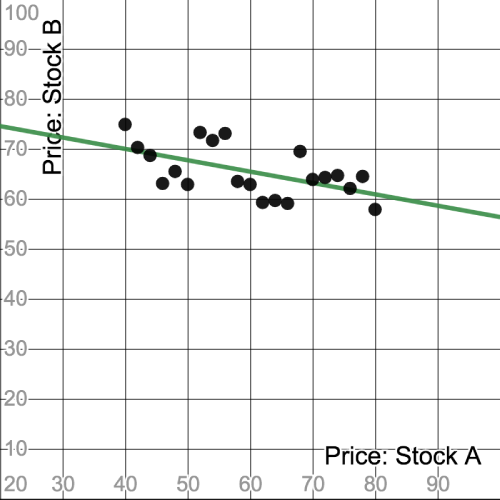Notice that the line of best fit (the green line) slants downward. This indicates a negative slope for the line. This means there is a negative correlation between the price of Stock A and the price of Stock B. In other words, as the price of Stock A goes up, the pierce of Stock B goes down.